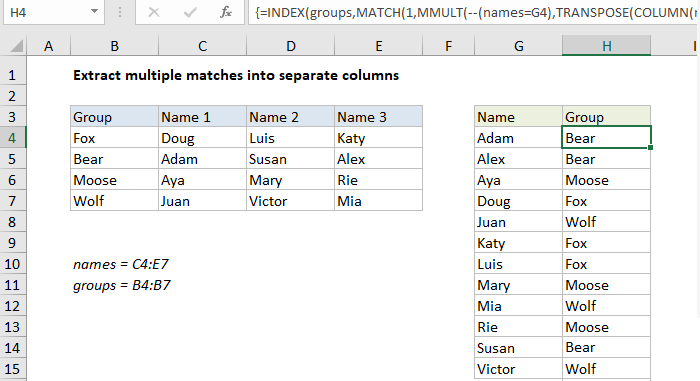## Excel Office

Excel How Tos, Tutorials, Tips & Tricks, Shortcuts

# Index and match on multiple columns in Excel

This tutorial shows how to calculate Index and match on multiple columns in Excel  using the example below;

## Formula

```{=INDEX(range1,MATCH(1,MMULT(--(range2=critera),
TRANSPOSE(COLUMN(range2)^0)),0))}```## Explanation

To lookup a value by matching across multiple columns, you can use an array formula based on the MMULT, TRANSPOSE, COLUMN, and INDEX. In the example shown, the formula in H4 is:

```{=INDEX(groups,MATCH(1,MMULT(--(names=G4),
TRANSPOSE(COLUMN(names)^0)),0))}```

where “names” is the named range C4:E7, and “groups” is the named range B4:B7. The formula returns the group that each name belongs to.

Note: this is an array formula and must be entered with control shift enter.

Also See:   How to check if cell contains one of many things in Excel

### How this formula works

Working from the inside out, the logical criteria used in this formula is:

`--(names=G4)`

where names is the named range C4:E7. This generates a TRUE / FALSE result for every value in data, and the double negative coerces the TRUE FALSE values to 1 and 0 to yield an array like this:

`{0,0,0;1,0,0;0,0,0;0,0,0}`

This array is 4 rows by 3 columns, matching the structure of “names”.

A second array is created with this expression:

`TRANSPOSE(COLUMN(names)^0))`

The COLUMN function is used to create a numeric array with 3 columns and 1 row, and TRANSPOSE converts this array to 1 column and 3 rows. Raising to the power of zero simply converts all numbers in the array to 1. The MMULT function is then used to perform matrix multiplication:

`MMULT({0,0,0;1,0,0;0,0,0;0,0,0},{1;1;1})`

and the resulting goes into the MATCH function as the array, with 1 as the lookup value:

`MATCH(1,{0;1;0;0},0)`

The MATCH function returns the position of the first match, which corresponds to the row of the first matching row meeting supplied criteria. This is feed into INDEX as the row number, with the named range “groups” as the array:

`=INDEX(groups,2)`

Finally, INDEX returns “Bear”, the group Adam belongs to.

Also See:   Count rows with multiple OR criteria in Excel

### Literal contains for criteria

To check for specific text values instead of an exact match, you can use the ISNUMBER and SEARCH functions together. For example, to match cells that contain “apple” you can use:

`=ISNUMBER(SEARCH("apple",data))`